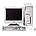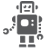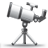# CSI input rate on IMX53

cancel
Showing results for
Show  only  | Search instead for
Did you mean:

## CSI input rate on IMX53

971 ViewsContributor V

Hi,

I wonder what is the real impact of bt mode on csi input rate:

In imx53RM manual at page 546, CSI input rate is said to be 135MP/s in BT1120 an d 67 MP/s in BT656, in 4:2:2.

Now at page 2633 in IPU chapter, CSI input rate is said to be 45Mp/s in 4:2:2, whether the bt mode is, it seems.

So, what input rate is to be considered ? Is it really possible to reach 135 MP/s in on-the-fly processing with BT1120 ?

Christophe

5 Replies
600 ViewsNXP Employee

I recommend that you can consider to calculate the required frequency as the followings:

F = FH * FW * FPS * BI * DF

Where

• FH = frame height (in pixels)

• FW = Frame width (in pixels)

• fps = frame rate (frames per second)

• BI = typically 35% overhead, should be assumed as 1.35. The actual blanking

intervals are a parameter of the attached device.

• DF = data format, defines the number of cycles needed to send a single pixel.

The number of cycles needed to send a single pixel depends on the interface and the data

format.

Data format examples:

• YUV422 over 16 bit = 1 cycles/pixel

• RGB888 over 8 bit = 1 cycle/pixel

• RGB565 over 16 bit = 2 cycles/pixel

• Bayer/Generic data = 1 cycle/pixel

• YUV422 over 8 bit = 2 cycles/pixel

• BT.656, YUV422 format = 2 cycles/pixel

• BT.1120, YUV422 format = 1 cycle/pixel

600 ViewsContributor V

Now i see where comes the 1,5 factor: ~1/(1-0.35).

Thanks.

Christophe.

600 ViewsNXP Employee

Hello, Christophe:

600 ViewsNXP Employee

Hi Christophe,

The maximum transfer rate must take account the interface bit width.

For a YUV4:2:2 (16 bits) format used over a 16 bits interface, the bandwidth will be: 135M/1,5 = 90M Pixels/sec

For a YUV4:2:2 (16 bits) format used over a 8 bits interface, it will take 2 cycles per bit to perform a 16 bits value. The bandwidth will be: 135M/(1,5*2) = 45M Pixels/sec

Rgds

Rogerio

600 ViewsContributor V

Hi Rogerio,

I agree with you for  the secondth point, but not the first:

In 4:2:2, you receive (Y0,Cr0) at n and (Y1,Cb0) at n+1, so 2 samples of 8 bits at each clock period, packed into one 16 bits sample. Moreover, the spec says 135 MP/s in 4:2:2. So the bandwidth must be 135 MP/s or 270 Mo/s.

So could you explain the 1,5 factor.

Rgds,

Christophe.